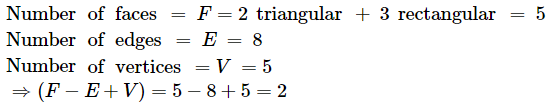# Verify Euler's relation for each of the following:

Question:

Verify Euler's relation for each of the following:

(i) A square

(ii) A tetrahedron

(iii) A triangular prism

(iv) A square pyramid

Solution:

Euler's relation is: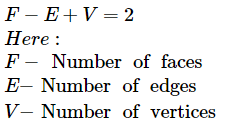(i) A square prism

(There is an error in this question. It should have been a square prism rather than square.)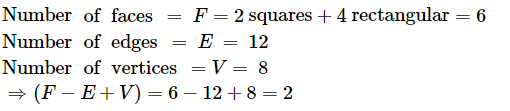(ii) A tetrahedron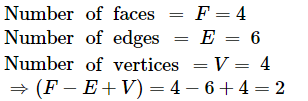(iii) A triangular prism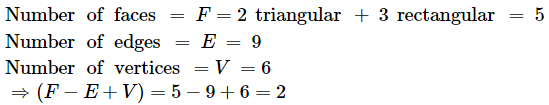(iv) A square pyramid